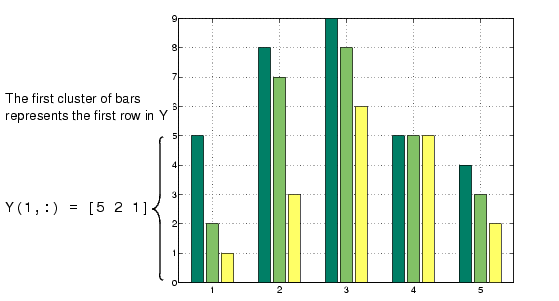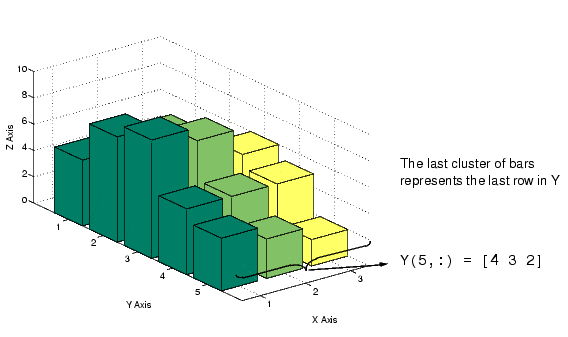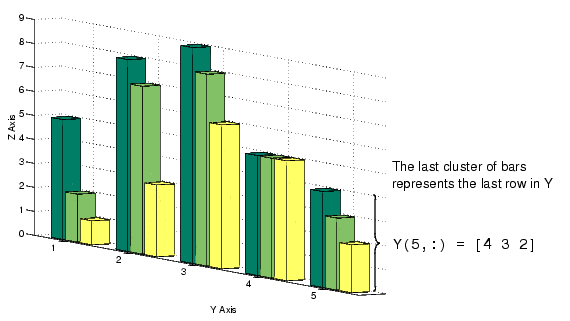GraphicsTypes of Bar Graphs

MATLAB has four specialized functions that display bar graphs. These functions display 2- and 3-D bar graphs, and vertical and horizontal bar graphs.

 Two-Dimensional Three-Dimensional Vertical `bar` `bar3` Horizontal `barh` `bar3h`

Grouped Bar Graph

By default, a bar graph represents each element in a matrix as one bar. Bars in a 2-D bar graph, created by the `bar` function, are distributed along the x-axis with each element in a column drawn at a different location. All elements in a row are clustered around the same location on the x-axis.

For example, define `Y` as a simple matrix and issue the `bar` statement in its simplest form.

• ```Y = [5 2 1
8 7 3
9 8 6
5 5 5
4 3 2];
bar(Y)
```

The bars are clustered together by rows and evenly distributed along the x-axis.Detached 3-D Bars

The `bar3` function, in its simplest form, draws each element as a separate 3-D block, with the elements of each column distributed along the y-axis. Bars that represent elements in the first column of the matrix are centered at `1` along the x-axis. Bars that represent elements in the last column of the matrix are centered at `size(Y,2)` along the x-axis. For example,

• ``````bar3(Y)
``````

displays five groups of three bars along the y-axis. Notice that larger bars obscure `Y(1,2)` and `Y(1,3)`.By default, `bar3` draws detached bars. The statement `bar3(Y,'detach')` has the same effect.

Labeling the Graph.   To add axes labels and x tick marks to this bar graph, use the statements

• ``````xlabel('X Axis')
```ylabel('Y Axis')
zlabel('Z Axis')
```set(gca,'XTick',[1 2 3])
``````

Grouped 3-D Bars

Cluster the bars from each row beside each other by specifying the argument `'group'.` For example,

• ``````bar3(Y,'group')
``````

groups the bars according to row and distributes the clusters evenly along the y-axis.Bar and Area Graphs Stacked Bar Graphs to Show Contributing Amounts© 1994-2005 The MathWorks, Inc.